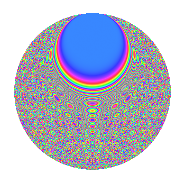# Properties

 Label 945.2.csLevel 945 Weight 2 Character orbit cs Rep. character $$\chi_{945}(104,\cdot)$$ Character field $$\Q(\zeta_{18})$$ Dimension 840 Newforms 2 Sturm bound 288 Trace bound 1

# Related objects

## Defining parameters

 Level: $$N$$ = $$945 = 3^{3} \cdot 5 \cdot 7$$ Weight: $$k$$ = $$2$$ Character orbit: $$[\chi]$$ = 945.cs (of order $$18$$ and degree $$6$$) Character conductor: $$\operatorname{cond}(\chi)$$ = $$945$$ Character field: $$\Q(\zeta_{18})$$ Newforms: $$2$$ Sturm bound: $$288$$ Trace bound: $$1$$ Distinguishing $$T_p$$: $$2$$

## Dimensions

The following table gives the dimensions of various subspaces of $$M_{2}(945, [\chi])$$.

Total New Old
Modular forms 888 888 0
Cusp forms 840 840 0
Eisenstein series 48 48 0

## Trace form

 $$840q - 24q^{4} - 30q^{9} + O(q^{10})$$ $$840q - 24q^{4} - 30q^{9} - 30q^{11} - 6q^{14} + 24q^{21} - 12q^{25} + 12q^{29} + 78q^{30} - 36q^{35} + 12q^{36} - 108q^{39} - 36q^{44} - 12q^{46} + 6q^{49} + 24q^{50} - 60q^{51} + 120q^{56} - 150q^{60} - 348q^{64} - 18q^{65} + 21q^{70} - 36q^{71} - 48q^{74} - 60q^{79} + 54q^{81} + 150q^{84} - 42q^{85} - 192q^{86} - 6q^{91} - 210q^{95} + 12q^{99} + O(q^{100})$$

## Decomposition of $$S_{2}^{\mathrm{new}}(945, [\chi])$$ into irreducible Hecke orbits

Label Dim. $$A$$ Field CM Traces $q$-expansion
$$a_2$$ $$a_3$$ $$a_5$$ $$a_7$$
945.2.cs.a $$24$$ $$7.546$$ $$\Q(\sqrt{-35})$$ $$0$$ $$0$$ $$0$$ $$0$$
945.2.cs.b $$816$$ $$7.546$$ None $$0$$ $$0$$ $$0$$ $$0$$